# Basics of Matrix, Vector, and various matrix operations

## Introduction

Linear Algebra is the field of mathematics concerned with vectors, matrices, and linear transforms. It creates a strong foundation for Machine learning and the implementation of algorithms in code.

We frequently face regression or classification problems in machine learning, and then error minimization techniques are used. We use linear algebra for these computations on large amounts of data.

These are the essential mathematical areas to understand the basic concepts of machine learning and deep learning.

## Matrix

The Matrix is a table of numbers arranged in rows and columns. It is like a 2-dimensional array. We write Dimensions of the Matrix as [ number of rows X number of columns ]

e.g.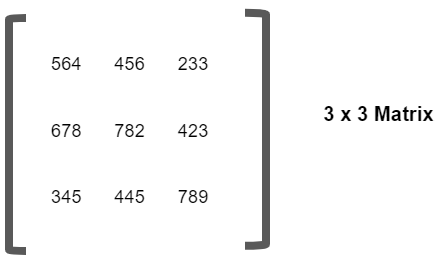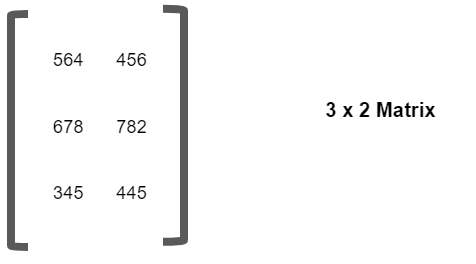Matrices of i x j dimensions can also be represented as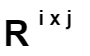.

Entries of a Matrix are represented by their row and column numbers. For example, We have a matrix A. Any element in this Matrix can be described as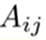, where i and j represent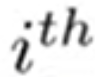row and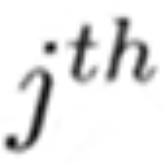column of the given element.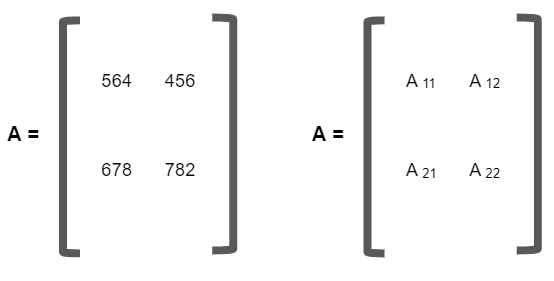Here,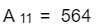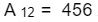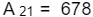## Vector

The vector is a particular case of a Matrix, having a single column.

It's an n x 1 Matrix.

E.g.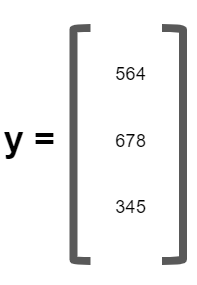Here is a 3-dimensional vector and can be represented as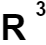.

To represent an element in a vector, we use the following notation: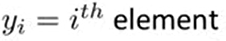( Here y1 = 546;  y2 = 678;  y3=345 )

Vectors can be 1-indexed and also 0-indexed unless specified. It's safe to use 1-indexed.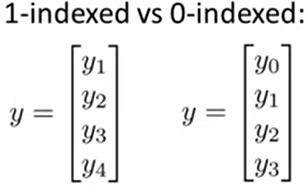Usually, uppercase letters are used to refer to Matrices, whereas lowercase letters are used for Vectors.

Elements of the first Matrix are added to the corresponding elements of the second Matrix by using row and column numbers. Only the same dimension matrices can be added, as the new Matrix formed will also have the exact dimensions.

E.g.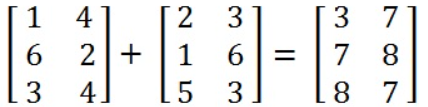## Scalar Multiplication

A scalar is just a real number that is multiplied by every element of the Matrix, one element at a time.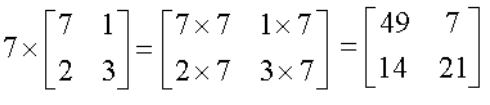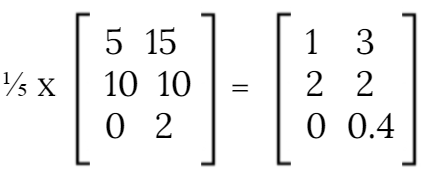## Matrix Multiplication

Matrix multiplication produces a resultant matrix from two matrices. The primary condition for matrix multiplication is that the number of columns of the first Matrix must be the same as the number of rows of the second Matrix. The resultant Matrix has the number of rows from the first and the number of columns from the second Matrix. The product of matrices and is denoted as XY.

Here we multiply each element inthe row of the first Matrix with the corresponding elements in thecolumn of the second Matrix and add them to obtain element ij of

the resultant Matrix. For example c1 = a1.b1 + a2.b2 + a3.b3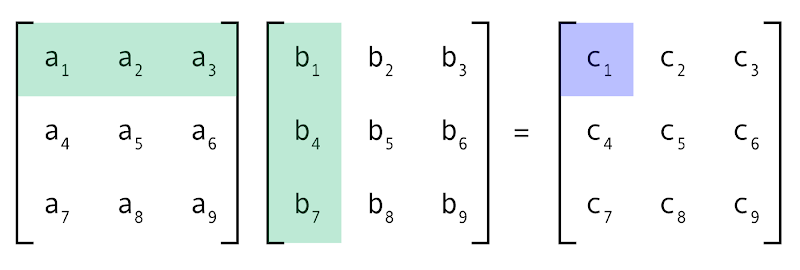Source: medium.com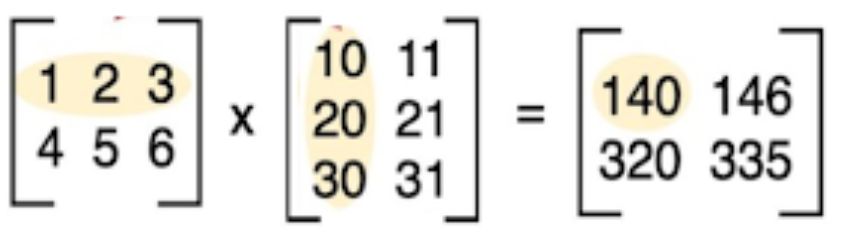## Multiplication with vector

To obtain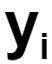, we multiply A’srow with elements of vector x and add them up.

The number of columns in Matrix A has to match with the number of rows in matrix B.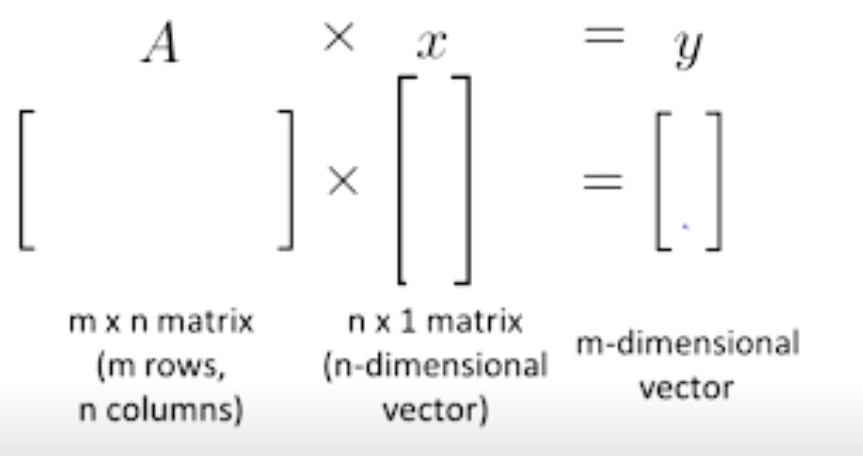## Matrix multiplication properties

1. Non-Commutative: Let A and B be matrices. Then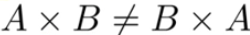.

E.g., We are getting different resultant matrices in the below cases.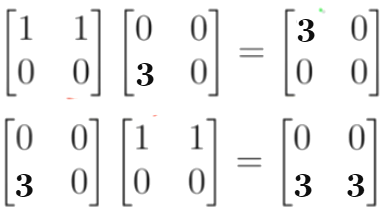2. Associative: Matrix multiplication is associative, Let A, B, C be three matrices.

Then A x (B x C) = (A x B) x C

## Identity Matrix

Identity Matrix of size n is defined as [ n x n ] square Matrix with 1's along the diagonal and 0's elsewhere. It is denoted by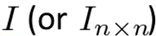.

For any matrix AA.I = I.A = A

Below are some examples of Identity matrices :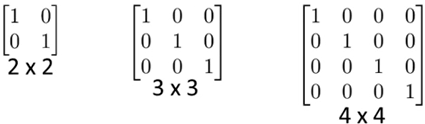## Diagonal Matrix

A diagonal matrix of size n is defined as [ n x n ] square Matrix with all the elements as 0 except those on the diagonal.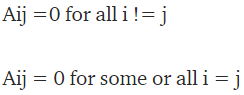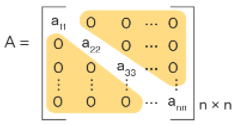Source: Cuemath

## Matrix Inverse operation

The inverse of a matrix is another matrix that results in an identity matrix on multiplication with the given Matrix. Not all numbers have an inverse, i.e., 0^-1 does not exist; Similarly, not all matrices have an Inverse. Matrices that do not have an inverse are "singular" or "degenerate" matrices.

If A is an [m x m] matrix and if it has an inverse,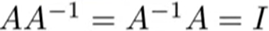.

E.g.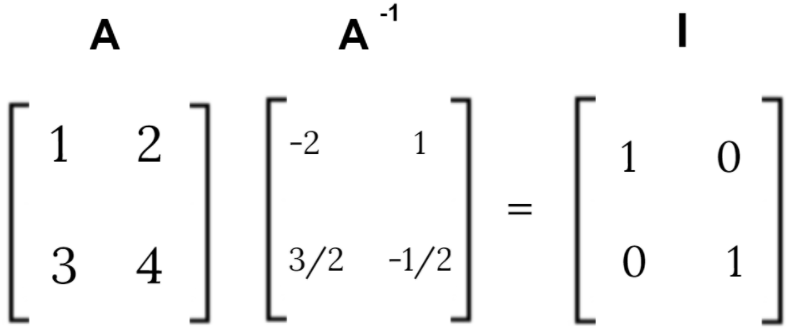## Matrix Transpose operation

Transpose of a Matrix is denoted by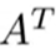. Let be an [m x n] Matrix and let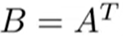. Then is an [n x m] Matrix where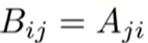. Transpose of a Matrix is similar to mirroring the Matrix over the diagonal axis.

E.g.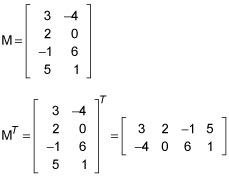Source: dummies.com

## A simple application of the above concepts in ML

Let’s assume we have three competing hypotheses: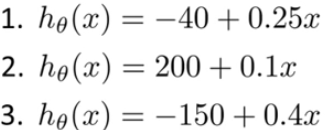And we need to test it for the following values of x:

2104

1416

1534

852

It can be done very efficiently by creating matrices for x and hypothesis and using matrix multiplication.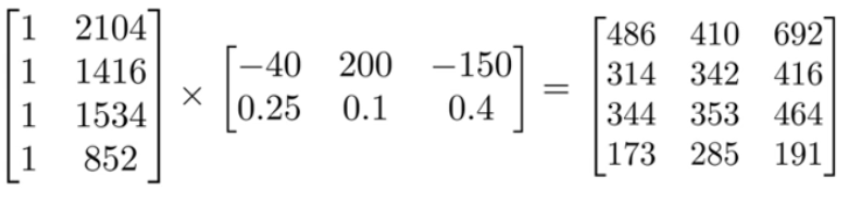Source: Linear algebra review by Andrew ng

1. Is non-square Matrix Invertible?
Non-square matrices (m x n matrix for which m ≠ n) do not have an inverse, But in some cases, such matrices might have a left inverse or right inverse.

2. Is Linear algebra required for machine learning?
It is not necessary to learn linear algebra before getting started with machine learning; However, It is advisable to learn linear algebra if you wish to get a better and deep understanding of machine learning algorithms and their applications.

3. What are the applications of linear algebra in computer science?
Below are some basic applications of linear algebra in computer science:
(i)Image Processing
(ii)Cryptography
(iii)Machine learning
(iv)Computer vision
(v)Optimizations
(vi)Graph-Algorithms and many more.

## Key takeaways

In this article, we have explored the basic Linear Algebra and Basics of Matrix, Vector, and various matrix operations for Machine Learning. I hope you are clear with all the material which is present in this article.

Linear algebra plays a key role in machine learning algorithms due to vectors, matrices, and several operations to handle vectors. Linear algebra can handle a large amount of data, or we can say, "linear algebra is the basic mathematics of data.". I hope this article was helpful.